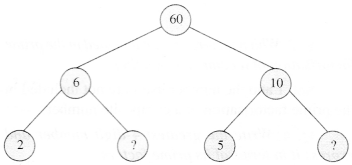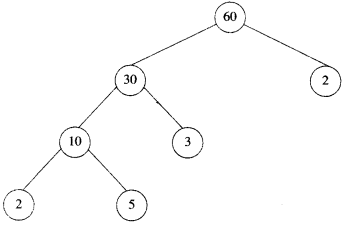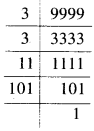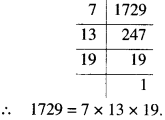NCERT Solutions for Class 6 Maths Chapter 3 Playing With Numbers Ex 3.5 are part of NCERT Solutions for Class 6 Maths. Here we have given NCERT Solutions for Class 6 Maths Chapter 3 Playing With Numbers Ex 3.5.

 Board CBSE Textbook NCERT Class Class 6 Subject Maths Chapter Chapter 3 Chapter Name Playing With Numbers Exercise Ex 3.6 Number of Questions Solved 12 Category NCERT Solutions

## NCERT Solutions for Class 6 Maths Chapter 3 Playing With Numbers Ex 3.5

Question 1.
Which of the following statements are true?
(a) If a number is divisible by 3, it must be divisible by 9.
(b) If a number is divisible by 9, it must be divisible by 3.
(c) A number is divisible by 18 if it is divisible by both 3 and 6.
(d) If a number is divisible by 9 and 10 both, then it must be divisible by 90.
(e) If two numbers are co-primes, at least one of them must be prime.
(f) All numbers which are divisible by 4 must be divisible by 8.
(g) All numbers which are divisible by 8 must also be divisible by 4.
(h) If a number exactly divides two numbers separately, it must exactly divide their sum.
(i) If a number exactly divides the sum of two numbers, it must exactly divide the two numbers separately.
Solution :
(a) This statement is false.
(b) This statement is true.
(c) This statement is false.
(d) This statement is true.
(e) This statement is false.
(j) This statement is false.
(g) This statement is true.
(h) This statement is true.
(i) This statement is false.

Question 2.
Here are two different factor trees for 60. Write the missing numbers.
(a)(b)Solution :
(a)(b)Question 3.
Which factors are not included in the prime factorization of a composite number?
Solution :
1 and the number itself are not included in the prime factorization of a composite number.

Question 4.
Write the greatest 4-digit number and e×press it in terms of its prime factors.
Solution :
The greatest 4 digit number is 9999.∴ 9999 = 3×3× 11 × 101.

Question 5.
Write the smallest 5-digit number and e×press it into the form of its prime factors.
Solution :
The smallest 5-digit number is 10000.∴ 10000 = 2×2×2×2×5×5×5×5.

Question 6.
Find all the prime factors of 1729 and arrange them in ascending order. Now state the relation, if any; between two consecutive prime factors.
Solution :All the prime factors of 1729 are 7, 13 and 19. When arranged in ascending order, these are 7, 13, 19. We observe that 13 – 7 = 6 19 – 13 = 6
Relation: The difference between two consecutive prime factors is 6.

Question 7.
The product of three consecutive numbers is always divisible by 6. E×plain this statement with the help of some examples.
Solution :
Example 1: Take three consecutive numbers 21, 22 and 23.
21 is divisible by 3.
22 is divisible by 2.
∴ 21 × 22 is divisible by 3 × 2 ( = 6)
∴ 21 × 22 × 23 is divisible by 6.

E×ample 2: Take three consecutive numbers 47, 48 and 49.
48 is divisible by 2 and 3 both.
∴ 48 is divisible by 2 × 3 (= 6)
47 × 48 × 49 is divisible by 6.

Question 8.
The sum of two consecutive odd numbers is divisible by 4. Verify this statement with the help of some examples.
Solution :
Example 1: Take two consecutive odd numbers 5 and 7.
Sum of these numbers = 5 + 7=12 12 is divisible by 4.
Example 2: 13 and 15
Sum of 13 and 15 =13+15 = 28
28 is divisible by 4.

Question 9.
In which of the following expressions, prime ffactorizationhas been done: j
(a) 24 = 2 × 3 × 4
(b) 56 = 7 × 2 × 2 × 2
(c) 70 = 2 × 5 × 7
(d) 54 = 2 × 3 × 9
Solution :
(a) Prime factorisation has not been done.
(b) Prime factorisation has been done.
(c) Prime factorisation has been done.
(d) Prime factorisation has not been done.

Question 10.
Determine, if 25110 is divisible by 45. [Hint : 5 and 9 are co-prime numbers. Test the divisibility of the number by 5 and 9]
Solution :
Divisibility of 25110 by 5
Number in the unit’s place of 25110 = 0
∴ 25110 is divisible by 5.
Divisibility of 25110 by 9
Sum of the digits of the number 25110 = 2+ 5 + 1 + 1 + 0 = 9
9 is divisible by 9.
∴ 25110 is divisible by 9
As 25110 is divisible by 5 and 9 both and 5 and 9 are co-prime numbers, so 25110 is divisible by 5 × 9 = 45.

Question 11.
18 is divisible by both 2 and 3. It is also divisible by 2 × 3 = 6. Similarly, a number is divisible by both 4 and 6. Can we say that the number must also be divisible by 4 × 6 = 24 ? If not, give an example to justify your answer.
Solution :
No we cannot say that the number will be divisible by 4 × 6 = 24, if it is divisible by both 4 and 6 because 4 and 6 are not co-prime numbers (they have two common factors 1 and 2).
Example : 36 is divisible by both 4 and 6.
But, 36 is not divisible by 24.

Question 12.
am the smallest number, having four different prime factors. Can you find me ?
Solution :
The smallest four different prime numbers are 2. 3. 5 and 7.
∴ The smallest number, having four different prime factors is 2 × 3 × 5 × 7 = 210.

We hope the NCERT Solutions for Class 6 Maths Chapter 3 Playing With Numbers Ex 3.5 help you. If you have any query regarding NCERT Solutions for Class 6 Maths Chapter 3 Playing With Numbers Ex 3.5, drop a comment below and we will get back to you at the earliest.